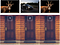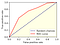# The Monty Hall Problem will drive you crazy

## Solving the famous brain teaser by math, statistics, and Monte Carlo methodsDoor by Sophie Dale, Goat by Peter Neumann, Car by Jp Valery on Unsplash and edited by me

# ROC Curve Transforms the Way We Look at a Classification Problem

## There is no machine learning algorithm that works best for all the problemsAn example of an ROC curve and AUC. Source: Huy Bui

## Motivation

`from sklearn.datasets import load_breast_cancerimport pandas as pddata=load_breast_cancer()columns=data.feature_namesX=pd.DataFrame(data.data, columns=columns)y=pd.DataFrame(data.target, columns=['Target'])df=pd.concat([y,X],axis=1)`

# Table of ContentThe Bayesian boy